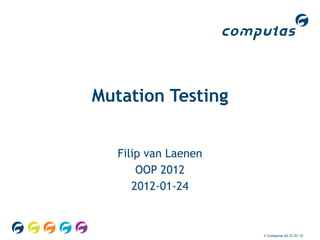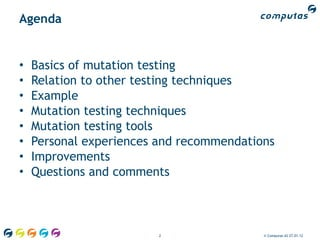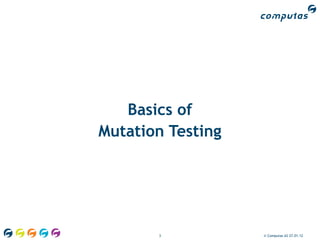Successfully reported this slideshow.

# Mutation testing (OOP 2012, 2012-JAN-24)

3

Share×
1 of 79
1 of 79

# Mutation testing (OOP 2012, 2012-JAN-24)

3

Share

My presentation about mutation testing at the OOP 2012 conference in Munich, Germany on 24 Januray 2012.

Notes:
* PIT now integrates with TestNG too

My presentation about mutation testing at the OOP 2012 conference in Munich, Germany on 24 Januray 2012.

Notes:
* PIT now integrates with TestNG too

## More Related Content

### Related Books

Free with a 14 day trial from Scribd

See all

### Related Audiobooks

Free with a 14 day trial from Scribd

See all

### Mutation testing (OOP 2012, 2012-JAN-24)

1. 1. Mutation Testing Filip van Laenen OOP 2012 2012-01-24 © Computas AS 27.01.12
2. 2. Agenda • Basics of mutation testing • Relation to other testing techniques • Example • Mutation testing techniques • Mutation testing tools • Personal experiences and recommendations • Improvements • Questions and comments 2 © Computas AS 27.01.12
3. 3. Basics of Mutation Testing 3 © Computas AS 27.01.12
4. 4. Mutation Testing in a Nutshell Seeking The Summoner @ The Daily WTF http://thedailywtf.com/Articles/Seeking-The-Summoner.aspx 4 © Computas AS 27.01.12
5. 5. Mutation Testing in a Nutshell (cont'd) 5 © Computas AS 27.01.12
6. 6. Mutation Testing in a Nutshell (cont'd) 6 © Computas AS 27.01.12
7. 7. Mutation Testing in a Nutshell (cont'd) • Unit tests guard the source code • But who guards the guardians? • Do the unit tests cover all source code? • Lines? • Branches? • Paths? • Do the unit tests test the right things? Mutation testing tests the tests! 7 © Computas AS 27.01.12
8. 8. Mutation Testing in a Nutshell (cont'd) 8 © Computas AS 27.01.12
9. 9. Mutation Testing in a Nutshell (cont'd) 9 © Computas AS 27.01.12
10. 10. Mutation Testing in a Nutshell (cont'd) 10 © Computas AS 27.01.12
11. 11. Mutation Testing in a Nutshell (cont'd) 11 © Computas AS 27.01.12
12. 12. Mutation Testing in a Nutshell (cont'd) 12 © Computas AS 27.01.12
13. 13. Mutation Testing in a Nutshell (cont'd) 13 © Computas AS 27.01.12
14. 14. Mutation Testing in a Nutshell (cont'd) def max(a, b) { return (a < b) ? b : a; } def max(a, b) { return (a ≤ b) ? b : a; } 14 © Computas AS 27.01.12
15. 15. Mutation Testing in a Nutshell (cont'd) 15 © Computas AS 27.01.12
16. 16. Does Mutation Testing Work? “ In practice, if the software contains a fault, there will usually be a set of mutants that can only be killed by a test case that also detects that fault. Geist et. al., “Estimation and Enhancement of Real-time Software Reliability through Mutation Analysis,” 1992 16 © Computas AS 27.01.12
17. 17. Does Mutation Testing Work? (cont'd) “ Complex faults are coupled to simple faults in such a way that a test data set that detects all simple faults in a program will detect most complex faults. K. Wah, “Fault Coupling in Finite Bijective Functions,” 1995 17 © Computas AS 27.01.12
18. 18. Does Mutation Testing Work? (cont'd) • “Generated mutants are similar to real faults.” • Andrews, Briand, Labiche, ICSE 2005 • “Mutation testing is more powerful than statement or branch coverage.” • Walsh, Ph.D. Thesis, State University of New York at Binghampton, 1985 • “Mutation testing is superior to data flow coverage criteria.” • Frankl, Weiss, Hu, Journal of Systems and Software, 1997 18 © Computas AS 27.01.12
19. 19. Relation to Other Testing Techniques 19 © Computas AS 27.01.12
20. 20. Relation to Other Testing Techniques • Unit tests • Test-Driven Development (TDD) • Test coverage • Static code analysis • Fuzz testing 20 © Computas AS 27.01.12
21. 21. Relation to Other Testing Techniques 21 © Computas AS 27.01.12
22. 22. Is Mutation Testing New? • R. Lipton, “Fault Diagnosis of Computer Programs,” 1971 • R. Lipton et. al., “Hints on Test Data Selection: Help for the Practicing Programmer,” 1978 • Historical obstacles: • No unit testing • No TDD • Time-consuming • No integration with IDEs 22 © Computas AS 27.01.12
23. 23. Practical Example of Mutation Testing 23 © Computas AS 27.01.12
24. 24. Practical Example # Returns the maximum of a. # @param a An array of integers. def max(a) { return …; } 24 © Computas AS 27.01.12
25. 25. Practical Example (cont'd) Omitted: max(null) Omitted: max([]) def max(a) { return …; } 25 © Computas AS 27.01.12
26. 26. Practical Example (cont'd) Assertion: max() = 0 def max(a) { return 0; } 26 © Computas AS 27.01.12
27. 27. Practical Example (cont'd) Assertion: max() = 0 Assertion: max() = 1 def max(a) { return 0; } 27 © Computas AS 27.01.12
28. 28. Practical Example (cont'd) Assertion: max() = 0 Assertion: max() = 1 def max(a) { return a.first; } 28 © Computas AS 27.01.12
29. 29. Practical Example (cont'd) Assertion: max() = 0 Assertion: max() = 1 Assertion: max([1, 2]) = 2 def max(a) { return a.first; } 29 © Computas AS 27.01.12
30. 30. Practical Example (cont'd) Assertion: max() = 0 Assertion: max() = 1 Assertion: max([1, 2]) = 2 def max(a) { nit t ests! m ← a.first; U age! cover foreach (e ∈ a) line 100% age? if (e > m) over nch c m ← e; 100% b ra return m; } 30 © Computas AS 27.01.12
31. 31. Practical Example (cont'd) Assertion: max() = 0 Assertion: max() = 1 Assertion: max([1, 2]) = 2 def max(a) { m ← a.first; foreach (e ∈ a) if (e > m) m ← e; return m; } 31 © Computas AS 27.01.12
32. 32. Practical Example (cont'd) Assertion: max() = 0 Assertion: max() = 1 Assertion: max([1, 2]) = 2 def max(a) { m ← a.first; foreach (e ∈ a) if (true) m ← e; return m; } 32 © Computas AS 27.01.12
33. 33. Practical Example (cont'd) Assertion: max() = 0 Assertion: max() = 1 Assertion: max([1, 2]) = 2 def max(a) { return a.last; } 33 © Computas AS 27.01.12
34. 34. Practical Example (cont'd) Assertion: max() = 0 Assertion: max() = 1 Assertion: max([1, 2]) = 2 Assertion: max([2, 1]) = 2 def max(a) { return a.last; } 34 © Computas AS 27.01.12
35. 35. Practical Example (cont'd) Assertion: max() = 0 Assertion: max() = 1 Assertion: max([1, 2]) = 2 Assertion: max([2, 1]) = 2 def max(a) { m ← a.first; foreach (e ∈ a) if (e > m) m ← e; return m; } 35 © Computas AS 27.01.12
36. 36. Practical Example (cont'd) Assertion: max() = 0 Assertion: max() = 1 Assertion: max([1, 2]) = 2 Assertion: max([2, 1]) = 2 def max(a) { m ← -∞; foreach (e ∈ a) if (e > m) m ← e; return m; } 36 © Computas AS 27.01.12
37. 37. Practical Example (cont'd) Assertion: max() = 0 Assertion: max() = 1 Assertion: max([1, 2]) = 2 def max(a) { m ← a.first; foreach (e ∈ a) if (e > m) ← Implicit else-branch! m ← e; return m; } 37 © Computas AS 27.01.12
38. 38. Practical Example (cont'd) Assertion: max() = 0 Assertion: max() = 1 Assertion: max([1, 2]) = 2 def max(a) { m ← -∞; foreach (e ∈ a) ? rage if (e > m) h cove ← Implicitcelse-branch! ran m ← e; 10 0% b return m; } 38 © Computas AS 27.01.12
39. 39. Practical Example (cont'd) • 100% test coverage may be illusory • Line coverage • Branch coverage • Path coverage • TDD principles easily broken • Even if you're very careful 39 © Computas AS 27.01.12
40. 40. Mutation Testing Techniques 40 © Computas AS 27.01.12
41. 41. Mutation Testing Techniques • Three aspects: • Mutation injection • Mutation types • Unit test selection per mutant • Key properties: • Efficiency • Performance 41 © Computas AS 27.01.12
42. 42. Mutation Injection • Source code mutation • Binary code mutation • Caveats: • De-mutation • Compilation errors • Invalid binary code 42 © Computas AS 27.01.12
43. 43. Mutation Types • Some mutations never change behaviour • Constants reused by unit tests • Log messages • Some mutations can change behaviour • Switching between < and ≠ • Switching between < and ≤ • Some mutations always change behaviour • Switching between < and ≥ 43 © Computas AS 27.01.12
44. 44. Mutation Types (cont'd) def max(a, b) { return (a < b) ? b : a; } def max(a, b) { return (a ≤ b) ? b : a; } def max(a, b) { return (a ≥ b) ? b : a; } 44 © Computas AS 27.01.12
45. 45. Mutation Types (cont'd) for (i ← 0; i < 10; i++) … for (i ← 0; i ≠ 10; i++) … for (i ← 0; i ≥ 10; i++) … 45 © Computas AS 27.01.12
46. 46. Mutation Types Guaranteed to Change Behaviour * • Negation of the comparison • Switching between = and ≠ • Switching between < and ≥ • Switching between > and ≤ • Negation of boolean conditions • Adding a ¬, ! or ~ • Shortcutting boolean conditions • Replacement with True or False 46 © Computas AS 27.01.12
47. 47. Unit Test Selection • Goal: find the unit test that “kills” the mutant • Selection aids: • Hints • Name/package matching • Code coverage tools • Automatic learning • Other heuristics 47 © Computas AS 27.01.12
48. 48. Unit Test Selection (cont'd) • System: • 50 classes • 20 unit tests per class • 1 ms per unit test • Unit testing time: 50 × 20 × 1ms = 1s • 10 mutants per class: • Brute-force: 10 × 50 × 1s = 6m 20s • Educated: 10 × 50 × 20 × 1ms = 10s 48 © Computas AS 27.01.12
49. 49. Unit Test Selection (cont'd) • System: • 500 classes • 20 unit tests per class • 1 ms per unit test • Unit testing time: 500 × 20 × 1ms = 10s • 10 mutants per class: • Brute-force: 10 × 500 × 10s = 13h 53m 20s • Educated: 10 × 500 × 20 × 1ms = 1m 40s 49 © Computas AS 27.01.12
50. 50. Complexity • f: Number of function points • φ: Number of function points per class, ≥ 1 • τ: Number of unit tests per function point, ≥ 1 • μ: Number of mutants per function point, ≥ 1 • Brute-force: (f × τ) × (f × μ) = τ × μ × f² • Educated force: τ × μ × φ × f • Ideal: τ × μ × f 50 © Computas AS 27.01.12
51. 51. Complexity (cont'd) • c: Number of classes • φ: Number of function points per class, ≥ 1 • τ: Number of unit tests per function point, ≥ 1 • μ: Number of mutants per function point, ≥ 1 • Brute-force: (f × τ) × (f × μ) = τ × μ × φ² × c² • Educated force: τ × μ × φ² × c • Ideal: τ × μ × φ × c 51 © Computas AS 27.01.12
52. 52. Loops for (i ← 0; i < 10; i++) … for (i ← 0; i < 10; i--) … 52 © Computas AS 27.01.12
53. 53. Infinite Loops • Terminate mutants that take too long to run • What's too long? • Ruins the performance • Can be hard to predict 53 © Computas AS 27.01.12
54. 54. Other Problems • Recursion: • Stack overflows • Out of memory exceptions • Syntax errors • Segmentation faults 54 © Computas AS 27.01.12
55. 55. Mutation Testing Tools 55 © Computas AS 27.01.12
56. 56. Mutation Testing Tools • Ruby: Heckle • Java: • Jester • Jumble • PIT • C#: Nester • Python: Pester 56 © Computas AS 27.01.12
57. 57. Heckle • Ruby • Test::Unit and rSpec • Usually run from the command-line • Runs a set of unit tests on a class or a method • Good to-the-point reporting • Good performance • Virtually no documentation • http://rubyforge.org/projects/seattlerb/ • http://docs.seattlerb.org/heckle/ 57 © Computas AS 27.01.12
58. 58. Heckle Mutations • Booleans • Numbers • Strings • Symbols • Ranges • Regexes • Branches (if, while, unless, until) 58 © Computas AS 27.01.12
59. 59. Heckle Sample Output Initial tests pass. Let's rumble. ***************************************************************** *** Greeter#greet loaded with 3 possible mutations ***************************************************************** 3 mutations remaining... 2 mutations remaining... 1 mutations remaining... No mutants survived. Cool! 59 © Computas AS 27.01.12
60. 60. My Heckle Sample Output filip@filip-laptop:~/github/wruf\$ rake heckle (in /home/filip/github/wruf) Doing mutation testing on 15 method(s) of FlickrSearcher against test/flickr_searcher_unit_test.rb: o FlickrSearcher#convert_photo_info [1/15] o FlickrSearcher#create_form_data_to_get_info_about_photo [2/15] o FlickrSearcher#create_form_data_to_get_info_about_user [3/15] o FlickrSearcher#create_form_data_to_search_photos [4/15] o FlickrSearcher#do_rest_request [5/15] o FlickrSearcher#get_author [6/15] … o FlickrSearcher#get_photo_info [12/15] o FlickrSearcher#get_photo_url [13/15] o FlickrSearcher#get_ref_url [14/15] o FlickrSearcher#get_ref_url_from_xml_photo_info [15/15] Checked 192 mutations, and no issues were found in FlickrSearcher. 60 © Computas AS 27.01.12
61. 61. My Heckle Sample Output (cont'd) Doing mutation testing on 7 method(s) of WrufSettings against test/wruf_settings_unit_test.rb: o WrufSettings#dimensions [1/7] o WrufSettings#dimensions= [2/7] o WrufSettings#hours [3/7] o WrufSettings#hours= [4/7] o WrufSettings#tags [5/7] o WrufSettings#tolerance [6/7] o WrufSettings#tolerance= [7/7] Checked 0 mutations, and no issues were found in WrufSettings. 61 © Computas AS 27.01.12
62. 62. Heckle Sample Report --- original +++ mutation def calculate_precision(rescaled_number) if (rescaled_number < 9.995) then return 2 else if (rescaled_number < 99.95) then return 1 else if (rescaled_number >= 99.95) then return 0 else - return -1 + return -70 end end end end 62 © Computas AS 27.01.12
63. 63. Jester • Java • JUnit • Usually run from the command-line • Grester for Maven2 • Operates on source code • Runs all unit tests on all classes • Reporting and documentation could be better • http://jester.sourceforge.net/ • http://sourceforge.net/projects/grester/ 63 © Computas AS 27.01.12
64. 64. Jester Sample Report Overview 64 © Computas AS 27.01.12
65. 65. Jester Sample Detailed Report 65 © Computas AS 27.01.12
66. 66. Pester and Nester • Pester • Jester for Python • PyUnit • Nester • Port of Jester for C# • NUnit • Integrated with Visual Studio • But outdated… • http://nester.sourceforge.net/ 66 © Computas AS 27.01.12
67. 67. Nester Sample Report 67 © Computas AS 27.01.12
68. 68. Jumble • Java • JUnit • Run from the command-line • Operates on byte code • Runs unit tests on a class • Reporting and documentation could be better • Claims to be faster than Jester • http://jumble.sourceforge.net/index.html 68 © Computas AS 27.01.12
69. 69. Jumble Sample Report Mutating Foo Tests: FooTest Mutation points = 12, unit test time limit 2.02s .. M FAIL: Foo:31: negated conditional M FAIL: Foo:33: negated conditional M FAIL: Foo:34: - -> + M FAIL: Foo:35: negated conditional ...... Score: 67% 69 © Computas AS 27.01.12
70. 70. PIT • Java • JUnit • Maven or command-line • Operates on byte code • Large set of mutators • Also possibly equivalent mutators available • Highly configurable • Sensible defaults • http://pitest.org/ 70 © Computas AS 27.01.12
71. 71. PIT Mutators • Conditionals Boundary • Negate Conditionals • Math • Increments • Invert Negatives • Inline Constant* • Return Values • Void Method Call • Non Void Method Call* • Constructor Call* 71 © Computas AS 27.01.12
72. 72. PIT Sample Report 72 © Computas AS 27.01.12
73. 73. Personal Experiences and Recommendations 73 © Computas AS 27.01.12
74. 74. Experiences and Recommendations • Use mutation testing from day 1 • Start on a small code base • Keep number of unit tests per class low • Have small classes • Select a good tool • Configurable • Flexible • One that can output the mutant 74 © Computas AS 27.01.12
75. 75. Experiences and Recommendations (cont'd) • Believe the tool • Or try to proof that the tool is wrong • Fix the problem • Don't turn mutation testing off • Embrace the “more than 100%” test coverage • Path coverage • Less code • More unit tests • More intelligent unit tests 75 © Computas AS 27.01.12
76. 76. Improvements 76 © Computas AS 27.01.12
77. 77. Improvements • Integration with more unit testing frameworks • Better unit test–source code mapping • Better heuristics • Parallellisation • Better reporting • IDE integration • Building tool integration 77 © Computas AS 27.01.12
78. 78. The Ideal Mutation Testing Tool™ • Easy integration with building tools • Easy integration with IDEs • Support for all unit testing frameworks • Human-aided unit test selection heuristics • Full parallellisation • Good source code mutation reporting 78 © Computas AS 27.01.12
79. 79. Questions? Contact: fvl@computas.com @filipvanlaenen Computas AS Tel +47-67 83 10 00 Lysaker Torg 45, pb 482 Fax +47-67 83 10 01 N-1327 Lysaker Org.nr: NO 986 352 325 MVA NORWAY www.computas.com 79 © Computas AS 27.01.12